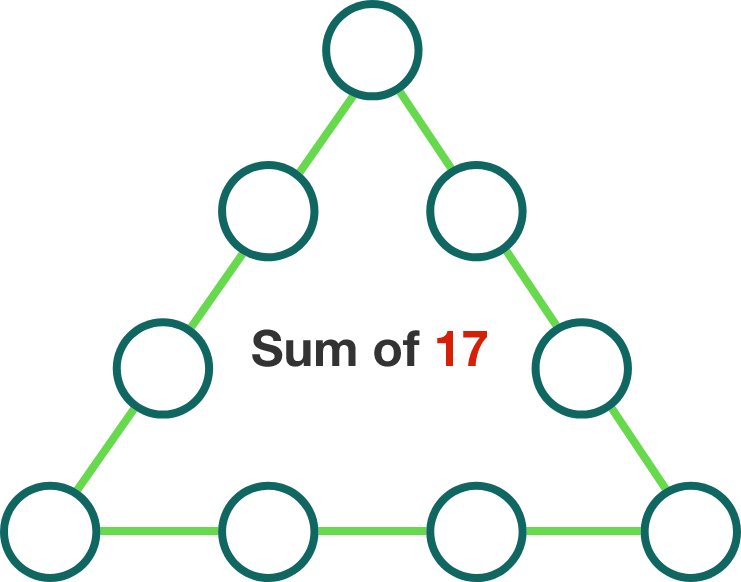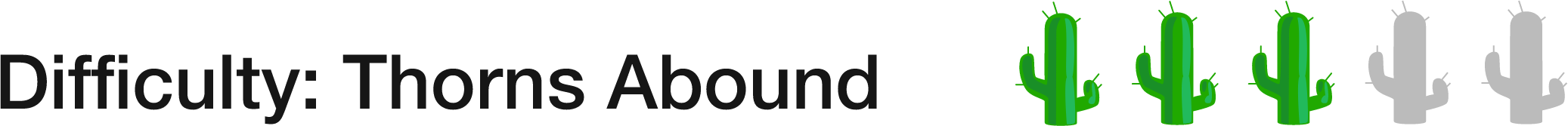# 34 of 100: Magic Triangle Perimeter

Logic Level 1Each of the integers from 1 to 9 is to be placed in one of the circles in the figure so that the sum of the integers along each side of the figure is 17. Determine the sum of the three integers placed in the corners.With this kind of puzzle it helps to start with either the largest or smallest digit, and figure out what happens after they get placed.

×